# Permanent magnet motor article

Do non-conservative potential perpetual running machines exist ?

Abstract:
It is shown that a system of coupled cog-wheels obeys a non-conservation relation of angular momentum.
It is shown shown by an example that a changing charge in a (conservative) ratched potential is subjected to a non-conservative force field which generates a perpetuum mobile cycle. It is shown that this model supports the perpetuum mobile claims of the Watson-Hartmann SMOT device only if it is assumed that magnetisation of the moving iron material depends from field gradient dH/dx as well. A nonlinear dependence of magnetisation from the field H alone gives no perpetuum mobile cycle. This fact is analogous to the known behaviour of electric cycles of non-linear capacity and coil.

1) Introduction
Because the positive messages grow in number that there exist already some working perpetuum mobile the question arises how this can be reconciliated with usual physical laws. The usual killer argument of physics against this claims is normally that all known fields can be described by potentials fields which conserve energy during one cycle in the field. Indeed, this argument gives a good hint where not to look for a perpetuum mobile, or which proposals will not work. But if we have a more precise look for not mentioned additional implicit assumptions laid down for energy conserving potentials we see quite easily that some these machines could run indeed.
Known to the author are the following implicit assumptions which must hold for conservation of energy and angular momentum additionally:

for angular momentum conservation:
If we have a composed system consisting of some (general) charges in a field (i.e. mass particles in gravitational fields, magnetic poles in magnetic field, electric charges in electric fields) the charges have to be either independent from the neighbour charge or the they have to be coupled to the neighbours by central forces .

for energy conservation:
1) the generating potential must be independent from additional variables as parameters, i.e. especially from time .
2) Charges in conservative field which generate the conservative force field by coupling to the field have to be constant or conserved.
We will see that if this assumptions must be dropped -because of the mechanic design of the construction- conservation laws break down and perpetuum mobiles become possible principally.

2) Angular moment non-conservation
It is well known fact that conservative force field do not allow any energy gain on any closed path in a potential field. For free moving systems angular momentum is conserved as well.However, this feature can be destroyed if we mount general charges in a general potential field on coupled cog wheeels or on similar working lever mechanisms. This is illustrated in fig.1 for two charges mounted on a cog wheel each. The radiuses of the wheel have the ratio n = r 1 /r 2 . For uncoupled charges (no cog wheel coupling) the torques on the one-dimensional periodic circular path of the charges can be expressed by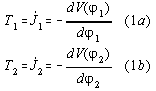whereare the angle position of the charge on the disk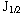are the angular momentum,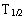is the torque and V is the potential of the(general) force field. For coupled wheels the equations for the torques areIt is interesting to multiply equation 2b) by n and to add it to equation 2a). We get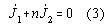This equation represents the "conservation of angular momentum" for this system. It is clear that this equations deviates from usual conservation of angular momentum.
However, the energy of this system is conserved. This is case because both equations fulfill the conditionas well. Therefore the system of equations can be written dependent only from one variable instead of two. Therefore, because the system is only one-dimensional, the potentials of both charges on the wheels can be obtained by integration, the system is conservative regarding energy and allows no energy gaining perpetuum mobile cycles.

3) Energy non-conservation
On an official level of science there exist publications about so called rachet potentials (periodic potential with an assymetric shape) dependent from time which allow to gain work from noise [1.] However, the practical application of these ideas is still at an rudimentary level.
On the other side, the newest claim of a successful perpetuum mobile permanent magnetic design is by Greg Watson  who got running on a circuit the linear patent proposal of Hartman. In the meantime this design has been successfully reproduced and works.
In this section we will present a simple one-dimensional mathematical model with a space dependent ratched potential which explains the working of Watson's SMOT,RMOD and variations therefrom quite naturally.
To get such a machine working we need
1) an assymetric shaped periodic, cyclic or linear, general potential field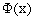(called ratched potential) which allows to derive a general conservative vector field E(x)= -d/dx therefrom.
2) a non-constant general charge q(x) dependent from path x coupling to the field E(x)
These ingredients allow us to build a cycle which work integral can be positive or negative depending on direction, which is indicative for perpetuum mobile action.
We illustrate this here by a very simple mathematical model example. We assume a charge which is dependent from x and obeys the relationWe assume a periodic ratched potential V(x) obeying the relation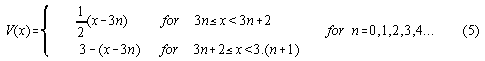Therefrom, the field E(x) can be derived according to the definition E(x)= -d/dx of the vector field of a potential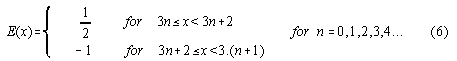The force F(x) of the field E on the charge q is F=q.E . Therefrom, the work of one cycle can be calculated to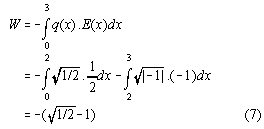We see here that W is negativ indicating a energy gain during a cycle. Fig.2 shows the potentialand the energy W gained versus x. Note that if the charge is chosen constant then W=0. The same would be probably the case if we choose a space dependent charge q(x) and any symmetric periodic potential V during the cycle, because then both function multiplied under the integral become symmetric and cancel to zero.
To be more practical and concrete we have to identify the mathematics with the physics. First, we test out a case of a isotropic nonlinear magnetic material with a unique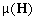which call the simple SMOT and which will not work -as we will see below:
If we move a magnetic permeable body in a magnetic field and neglect thermic losses the formula describing the energy conversion of mechanic energy into magnetic energy and vice versa iswhere M is the polarisation per volume element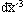and H is the magnetic H field and B is the magnetic B-field. The second equation holds because the added cycle integral including the vacuum polarisation term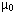H is zero.
Now, becauseis symmetric resp. to H=0,can be written in a Taylor expansion expanded around H=0 with the second order term as lowest order term.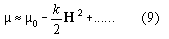with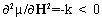and 0=const. being the initial permeability at H=0. Using the definition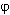:=1/2.H2 we rewrite (8) using (9)If the function in the circle integral is unique and does not depend from other parameters the circle integral coincides with a usual integral. Because of the periodicity of the function H(t,x') is the same before and after a cycle of the time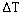, it follows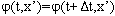and therefore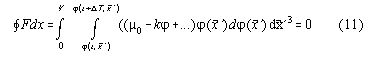The whole consideration can be extended to a ferrite with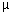changing with x' if eq.(9) holds locally. Therefore, in this respect the simple SMOT behaves formally completely analogous to other null-result problems arising in the electronic perpetuum mobile scene, i.e. the charge and discharge of an nonlinear time invariant coil or capacitance. This can be shown as follows:
According the theorem of Tellegen in a network of 2-pole element the energy conservation equation of power of the single elements holds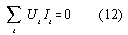The electronic elements may be taken quite arbitrary. These elements may be resistors, capacitors inductors and they even may include parts of more pole electronic components as a primary or secondary of a transformer for example.
In order to discuss the overunity capability of electronic components it is more convenient to write down the balance equation above in terms of energy and to restrict the discussion on cyclic electronic processes. Therefore we get the energy balance equation for a cyclic process of lengthNow we can distiguish the electronic components as energy source if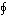U dQ < 0 and as electric consumer ifU dQ > 0. To illustrate things these cases can be represented as working areas of different orientation of the cycle.
Now, for people believing in overunity the questions arises whether passive electronic components can be brougth into a energy sucking state with negative electric work area when they act as electric energy sources contrary to the usual positive dissipating work area of a resistor for example. We will see that for any conventional non-linear time invariant components the result is zero. The energy of the electric cycle of a nonlinear capacitor is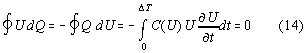An analog consideration can be done with an nonlinear inductivity because in this case holds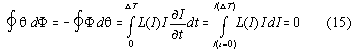where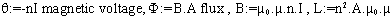inductivity.
In both cases (eq.14 and 15) the result is zero for a periodic cycle of timebecause U()=U(0) and I()=I(0).
It is clear that both cases are formally completely analogous to the SMOT as well, see Tab.1.
Therefore, some TEP-circuits proposed by us probably does not work as well and the effects are due to energy input through the parasitic capacitance of the FET. It should be noted that some of this facts are well known and can be found in textbooks of network theory.

Tab.1: analogy between SMOT and electric cycles with nonlinear inductivity and capacitance
for these system no perpetuum mobile action is possible

 system parametrisation energy 1 energy 2 simple SMOT path x magnetic field mechanical energy inductivity time t magnetic field electric current capacitance time t electric field electric current

Our result is contrary to the suggestion given in our last version of this SMOT article. There we overlooked the fact that in our model substanceis dependent from H and not from the field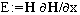which makes the big difference.
Therefore, the question arise whether cyclic non-conservative processes work can exist at all. We will show that the existence of cycles with overunity efficiency depends from the question whether a material can be found whose material constantdepends on a field likeas well.
In the most general case it holds the matrix equationas constitutive equation for any ferromagnetic material due to the field character of the electromagnetic theory. Therefore to run a SMOT  we need

1) material withdependent from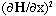2) an assymmetric ratched field H(x) from outside

Proof:
If an additional contribution due to this inhomogenity exists the formula for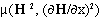can be expanded at H=0 and we get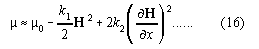With the definition:=H2/2 , and using eq.(8) we get finally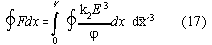This is a expression which is nonzero for nonsymmetric periodic functions of(x) in a cycle.Due to the theory of ferromagnetism by Landau  terms of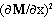are possible for the free energy potential. These terms are attributed to anisotropy of magnetisation caused by Bloch walls of the magnetic domains in the bulk. At the moment the author does not see clearly whether it is correct to apply this theory to the present problem and whether material can be designed appropriately by making the material grains of the bulk so very fine that the inhomogenity terms get quantitatively relevant. It should be noted that Bruce Vandeayrs  SMOT contained metallic powder as ferromagnetic material and another overunity claimed motor of Bill Mullers motor  does it as well.

4) Conclusion
If we regard mathematics every differential equation has its own conservation laws. It is clear that real systems and consequenly its equations describing the physics can be chosen the conservation law coinciding with the usual conservation laws of physics. But I think I have shown that the possibility of other choices exist as well, especially if the symmetries of fields are broken.

Bibliography:
1) T.W.B. Kibble  Classical Mechanics (second edition) p.127  Mc Graw Hill London 1973

4) E. Hartman U.S. Patent Nr.4215330 date 29.7.1980
look at the IBM patent server

5) epitaxy@mail.localaccess.com 20.6.97 at mailing list freenrg-l at www.eskimo.com/~billb
see as well at J.L. Naudin's server

6) Gary's magnetic motor
Harper's new monthly magazine March 1879, p.601-605

7) R. Unbehauen Grundlagen der Elektrotechnik 2 4.Aufl. Springer, Berlin 1994

8) L.D Landau, E.M. Lifshitz Elektrodynamik der Kontinua Vol.8
Lehrbuch der theoretischen Physik Akademie Verlag Berlin 1985

9) Gottfried Hilscher Energie für das 3.Jahrtausend VAP-Verlag Wiesbaden 1996

10) S.C. Anco, G. Bluman Phys. Rev. Lett. 78 (1997) p.2869-2873
Direct construction of conservation laws from field equationsFig.1: How to generate a system not-conserving angular momentum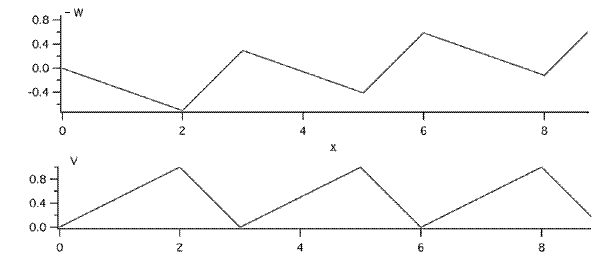Fig.2: Potential V versus x and work W versus x of a ratched potential ona non constant charge. Is is shown that the potential is strictly periodic -contrary to the work which rises from cycle to cycle, comp. text

>>>>>>>>>>>>>>>>>>>>>>> >>>>back to overunity-theory page<<<<<<<<<<<<<<<<<<<<<<<<<<<